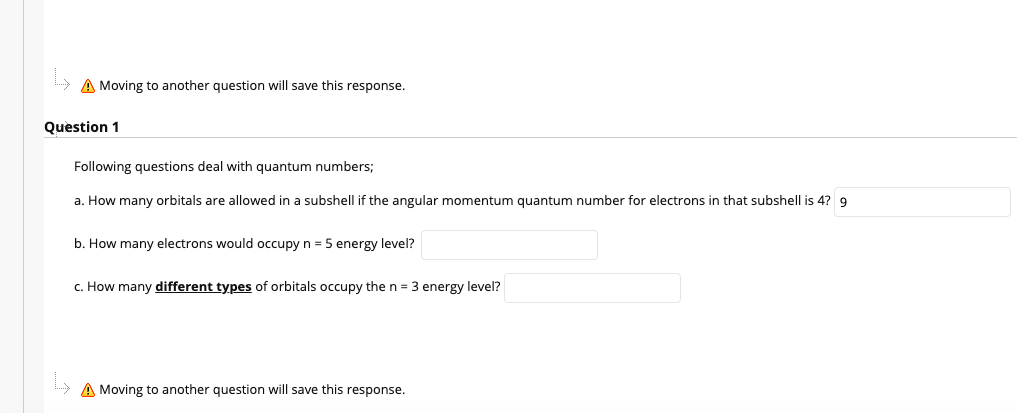# Following questions deal with quantum numbers; a. How many orbitals are allowed in a subshell if the angular momentum quantum number for electrons in the subshell is ℓ = 4? b. How many electrons would occupy n = 5 energy level? c. How many different types of orbitals occupy the n = 3 energy level?